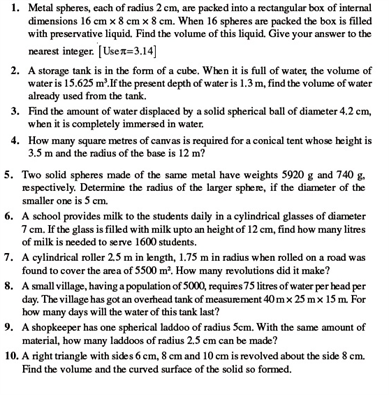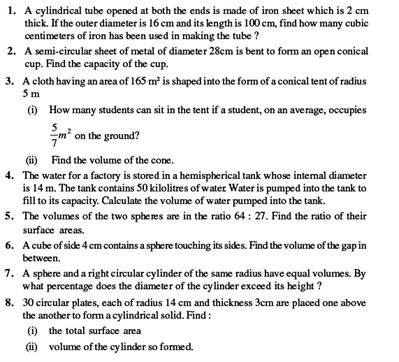# Revision Notes For CBSE Class 9 Math Chapter -13 Surface Areas and Volumes

An Area is defined as the total space occupied by a two-dimensional flat surface. It is mainly measured in square units. Total Surface Area and Curved Surface Area are two different types of an Area.

The sum of the areas of all the surfaces of a 3D shape is termed as the surface area.The surface area of a closed surface includes its length, width (w), and its height (h).

Volume is the measuring quantity which is mainly used to measure the amount of space occupied by a closed surface. Volume is  mainly measured in  the cubic metre.The formulas for calculating the Surface Areas and Volumes varies for different geometrical shapes.

Check here for the free CBSE Class 9 Math revision notes for Chapter 13- Surface Areas and Volumes.